Next: Example Longitude Calculations Up: The Sun Previous: The Sun

# Determination of Ecliptic Longitude

Our solar longitude model is sketched in Figure 21. From a geocentric point of view, the sun,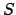, appears to execute a (counterclockwise) Keplerian orbit of major radius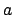, and eccentricity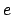, about the earth,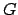. As has already been mentioned, the circle traced out by the sun on the celestial sphere is known as the ecliptic circle. This circle is inclined at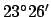to the celestial equator, which is the projection of the earth's equator onto the celestial sphere. Suppose that the angle subtended at the earth between the vernal equinox (i.e., the point at which the ecliptic crosses the celestial equator from south to north) and the sun's perigee (i.e., the point of closest approach to the earth) is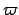. This angle is termed the longitude of the perigee, and is assumed to vary linearly with time: i.e.,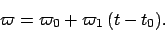(95)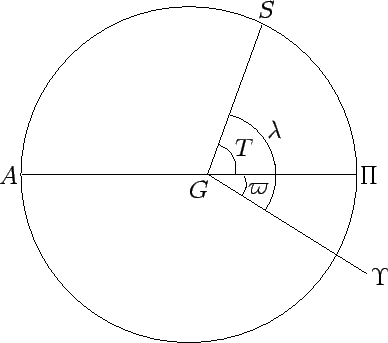The sun's ecliptic longitude is defined as the angle subtended at the earth between the vernal equinox and the sun. Hence, from Fig. 21,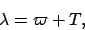(96)

whereis the true anomaly (see Cha. 4). By analogy, the mean longitude is written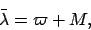(97)

where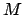is the mean anomaly (see Cha. 4). It follows from Eq. (82) that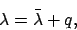(98)

where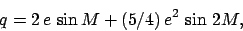(99)

is called the equation of center. Note that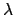,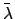,, andare usually written as angles in the rangeto, whereas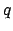is generally written as an angle in the range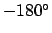to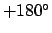.

The mean longitude increases uniformly with time (since bothandincrease uniformly with time) as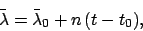(100)

where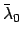is termed the mean longitude at epoch,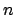the rate of motion in mean longitude, and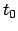the epoch. We can also write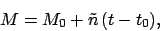(101)

where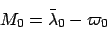(102)

is called the mean anomaly at epoch, and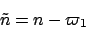(103)

the rate of motion in mean anomaly.

Our procedure for determining the ecliptic longitude of the sun is described below. The requisite orbital elements (i.e.,,,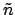,, and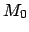) for the J2000 epoch (i.e., 12:00 UT on January 1, 2000 CE, which corresponds to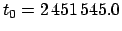JD) are listed in Table 30. These elements are calculated on the assumption that the vernal equinox precesses at the uniform rate of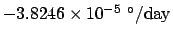. The ecliptic longitude of the sun is specified by the following formulae: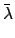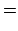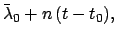(104)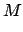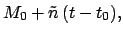(105)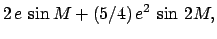(106)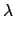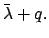(107)

These formulae are capable of matching NASA ephemeris data during the years 1995-2006 CE (see http://ssd.jpl.nasa.gov/) with a mean error of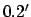and a maximum error of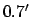.

The ecliptic longitude of the sun can be calculated with the aid of Tables 32 and 33. Table 32 allows the mean longitude,, and mean anomaly,, of the sun to be determined as functions of time. Table 33 specifies the equation of center,, as a function of the mean anomaly.

The procedure for using the tables is as follows:

1. Determine the fractional Julian day number,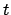, corresponding to the date and time at which the sun's ecliptic longitude is to be calculated with the aid of Tables 27-29. Form, whereis the epoch.
2. Enter Table 32 with the digit for each power of 10 in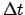and take out the corresponding values of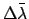and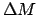. Ifis negative then the corresponding values are also negative. The value of the mean longitude,, is the sum of all thevalues plus the value ofat the epoch. Likewise, the value of the mean anomaly,, is the sum of all thevalues plus the value ofat the epoch. Add as many multiples oftoandas is required to make them both fall in the rangeto. Roundto the nearest degree.
3. Enter Table 33 with the value ofand take out the corresponding value of the equation of center,, and the radial anomaly,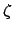. (The latter step is only necessary if the ecliptic longitude of the sun is to be used to determine that of a planet.) It is necessary to interpolate ifis odd.
4. The ecliptic longitude,, is the sum of the mean longitude,, and the equation of center,. If necessary, convertinto an angle in the rangeto. The decimal fraction can be converted into arc minutes using Table 31. Round to the nearest arc minute.
Two examples of the use of this procedure are given below.Next: Example Longitude Calculations Up: The Sun Previous: The Sun
Richard Fitzpatrick 2010-07-21